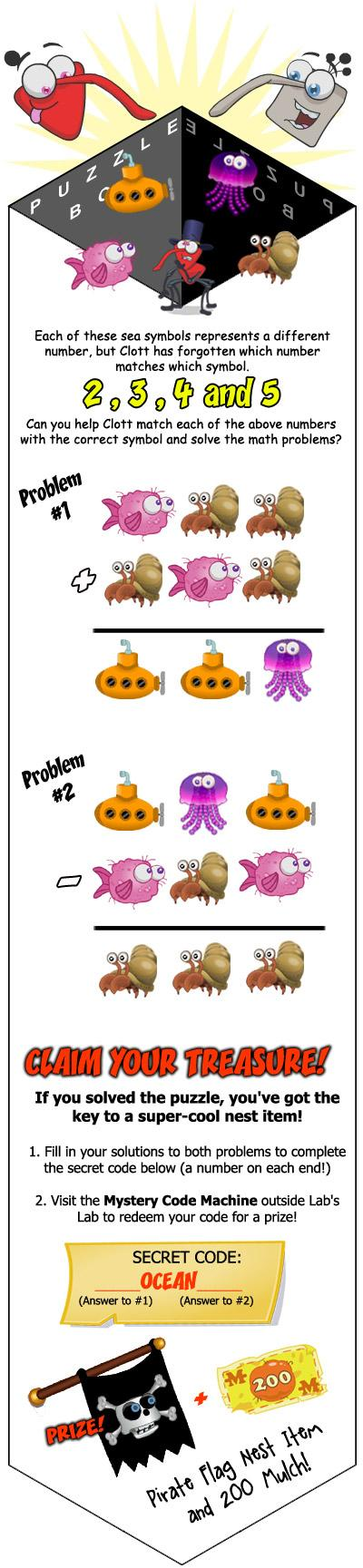# Weekend Puzzle Challenge – 15th January 2012

In Weevil Weekly, Scribbles said that if you want a Pirate Flag for your nest you need to do the Weekend Puzzle Challenge and Cheats Whiz is here to help!The code is simply 554OCEAN222 but lets look at how this problem is solved!

1. Let’s start with Problem 1! Crab add Crab equals Jellyfish. The numbers we have are 2, 3, 4, and 5. An even number plus an even number equals an even number and the number here is the same. It must be two add two equals four.
2. Now we know that crab is two and four is jellyfish, this should be easier. So crab add fish equals submarine. So we know that crab is two, and there is no jellyfish, fish and submarine must be either 3 or 5. Well five add 2 equals seven, we don’t have seven so fish must me three and submarine must be five. So it is two add three is five.
3. Exactly the same sum, numbers swapped round. So three add two equals five.
4. Okay, so answer to Problem 1 is 554!
5. Now we know what all the symbols stand for so this is pips. For Problem 2 the answer is crab, crab, crab. We know that crab is two so the answer to Problem 2 is 222.
6. Turn it into the code, it is 554OCEAN222

Could you figure this out on your own? Is this your favourite type of Weekend Puzzle Challenge? Do you adore your new Pirate Flag? Let us know in the comments!